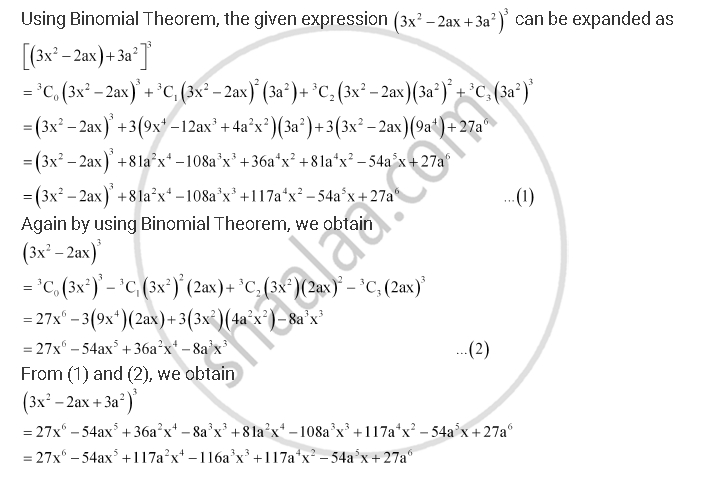CBSE (Arts) Class 11CBSE
Share

Books Shortlist

Find the Expansion of (3x2 – 2ax + 3a2)3 Using Binomial Theorem. - CBSE (Arts) Class 11 - Mathematics

ConceptBinomial Theorem for Positive Integral Indices

Question

Find the expansion of (3x2 – 2ax + 3a2)3 using binomial theorem.

SolutionIs there an error in this question or solution?

APPEARS IN

Solution Find the Expansion of (3x2 – 2ax + 3a2)3 Using Binomial Theorem. Concept: Binomial Theorem for Positive Integral Indices.
S